This document is part of the HTML publication "An Introduction to the Imperative Part of C++"

The original version was produced by Rob Miller at Imperial College London, September 1996.

Version 1.1 (modified by David Clark at Imperial College London, September 1997)

Version 1.2 (modified by Bob White at Imperial College London, September 1998)

Version 1.3, 1.4, 2.0, ..., 2.21, 3.0 (modified by William Knottenbelt at Imperial College London, September 1999-September 2023)

# 7. Pointers

## 7.1 Introducing Pointers

In the previous lectures, we have not given many methods to control the amount of memory used in a program. In particular, in all of the programs we have looked at so far, a certain amount of memory is reserved for each declared variable at compilation time, and this memory is retained for the variable as long as the program or block in which the variable is defined is active. In this lecture we introduce the notion of a pointer, which gives the programmer a greater level of control over the way the program allocates and de-allocates memory during its execution.

### Declaring Pointers

A pointer is just the memory address of a variable, so that a pointer variable is just a variable in which we can store different memory addresses. Pointer variables are declared using a "*", and have data types like the other variables we have seen. For example, the declaration

```	int *number_ptr;
```

states that "number_ptr" is a pointer variable that can store addresses of variables of data type "int". A useful alternative way to declare pointers is using a "typedef" construct. For example, if we include the statement:

```	typedef int *IntPtrType;
```

we can then go on to declare several pointer variables in one line, without the need to prefix each with a "*":

```	IntPtrType number_ptr1, number_ptr2, number_ptr3;
```

### Assignments with Pointers Using the Operators "*" and "&"

Given a particular data type, such as "int", we can write assignment statements involving both ordinary variables and pointer variables of this data type using the dereference operator "*" and the (complementary) address-of operator "&". Roughly speaking, "*" means "the variable located at the address", and "&" means "the address of the variable". We can illustrate the uses of these operators with a simple example program:

```	#include <iostream>
using namespace std;

typedef int *IntPtrType;

int main()
{
IntPtrType ptr_a, ptr_b;
int num_c = 4, num_d = 7;

ptr_a = &num_c;              /* LINE 10 */
ptr_b = ptr_a;                   /* LINE 11 */

cout << *ptr_a << " " << *ptr_b << "\n";

ptr_b = &num_d;              /* LINE 15 */

cout << *ptr_a << " " << *ptr_b << "\n";

*ptr_a = *ptr_b;                 /* LINE 19 */

cout << *ptr_a << " " << *ptr_b << "\n";

cout << num_c << " " << *&*&*&num_c << "\n";

return 0;
}
```

Program 7.1.1

The output of this program is:

```	4 4
4 7
7 7
7 7
```

Diagramatically, the state of the program after the assignments at lines 10 and 11 is: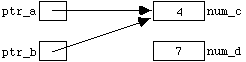after the assignment at line 15 this changes to: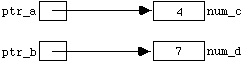and after the assignment at line 19 it becomes: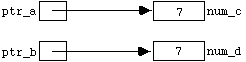Note that "*" and "&" are in a certain sense complementary operations; "*&*&*&num_c" is simply "num_c".

### The "new" and "delete" operators, and the constant "NULL".

In Program 7.1.1, the assignment statement

```	ptr_a = &num_c;
```

(in line 10) effectively gives an alternative name to the variable "num_c", which can now also be referred to as "*ptr_a". As we shall see, it is often convenient (in terms of memory management) to use dynamic variables in programs. These variables have no independent identifiers, and so can only be referred to by dereferenced pointer variables such as "*ptr_a" and "*ptr_b".

Dynamic variables are "created" using the reserved word "new", and "destroyed" (thus freeing-up memory for other uses) using the reserved word "delete". Below is a program analogous to Program 7.1.1, which illustrates the use of these operations:

```	#include <iostream>
using namespace std;

typedef int *IntPtrType;

int main()
{
IntPtrType ptr_a, ptr_b;    /* LINE 7 */

ptr_a = new int;            /* LINE 9 */
*ptr_a = 4;
ptr_b = ptr_a;              /* LINE 11 */

cout << *ptr_a << " " << *ptr_b << "\n";

ptr_b = new int;            /* LINE 15 */
*ptr_b = 7;                 /* LINE 16 */

cout << *ptr_a << " " << *ptr_b << "\n";

delete ptr_a;
ptr_a = ptr_b;              /* LINE 21 */

cout << *ptr_a << " " << *ptr_b << "\n";

delete ptr_a;               /* LINE 25 */

return 0;
}
```

Program 7.1.2

The output of this program is:

```	4 4
4 7
7 7
```

The state of the program after the declarations in line 7 is: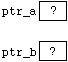after the assignments in lines 9, 10 and 11 this changes to: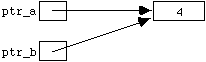after the assignments at lines 15 and 16 the state is: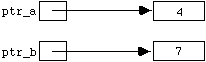and after the assignment at line 21 it becomes: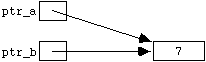Finally, after the "delete" statement in lines 25, the program state returns to:In the first and last diagrams above, the pointers "ptr_a" and "ptr_b" are said to be dangling. Note that "ptr_b" is dangling at the end of the program even though it has not been explicitly included in a "delete" statement.

If "ptr" is a dangling pointer, use of the corresponding dereferenced expression "*ptr" produces unpredictable (and sometimes disastrous) results. Unfortunately, C++ does not provide any inbuilt mechanisms to check for dangling pointers. However, safeguards can be added to a program using the special symbolic memory address "NULL". Any pointer of any data type can be set to "NULL". For example, if we planned to extend Program 7.1.2 and wanted to safeguard against inappropriate use of the dereferenced pointer identifiers "*ptr_a" and "*ptr_b", we could add code as follows:

```	#include <iostream>
...
...
delete ptr_a;
ptr_a = NULL;
ptr_b = NULL;
...
...
if (ptr_a != NULL)
{
*ptr_a = ...
...
...
```

Fragment of Program 7.1.3

Please note that the latest edition of the C++ standard (C++11) introduces the constant nullptr alongside NULL. Support for this is gradually being introduced into compilers. You may be interested to read an explanation of the subtle rationale behind the introduction of nullptr.

In the case that there is not sufficient memory to create a dynamic variable of the appropriate data type after a call to "new", modern C++ implementations throw an exception called std::bad_alloc which can be caught by the programmer using a try ... catch block. Alternatively, by a call to "new (nothrow)" C++ can be made to set the corresponding pointer to "NULL" in the case of allocation failure. The following code typifies the kinds of safety measure that might be included in a program using dynamic variables:

```	#include <iostream>
#include <cstdlib>   /* ("exit()" is defined in <cstdlib>) */
...
using namespace std;
...
try {
ptr_a = new int;
cout << "Sorry, ran out of memory";
exit(1);
}
...
ptr_a = new (nothrow) int;
if (ptr_a == NULL)
{
cout << "Sorry, ran out of memory";
exit(1);
}
...
...
```

Fragment of Program 7.1.4

Pointers can be used in the standard way as function parameters, so it would be even better to package up this code in a function:

```	void assign_new_int(IntPtrType &ptr)
{
ptr = new (nothrow) int;
if (ptr == NULL)
{
cout << "Sorry, ran out of memory";
exit(1);
}
}
```

Fragment of Program 7.1.5

## 7.2 Array Variables and Pointer Arithmetic

In the last lecture we saw how to declare groups of variables called arrays. By adding the statement

```	int hours;
```

we could then use the identifiers

```	hours   hours   hours   hours   hours   hours
```

as though each referred to a separate variable. In fact, C++ implements arrays simply by regarding array identifiers such as "hours" as pointers. Thus if we add the integer pointer declaration

```	int *ptr;
```

to the same program, it is now perfectly legal to follow this by the assignment

```	ptr = hours;
```

After the execution of this statement, both "ptr" and "hours" point to the integer variable referred to as "hours". Thus "hours", "*hours", and "*ptr" are now all different names for the same variable. The variables "hours", "hours", etc. now also have alternative names. We can refer to them either as

```	*(hours + 1)   *(hours + 2)   ...
```

or as

```	*(ptr + 1)   *(ptr + 2)   ...
```

In this case, the "+ 2" is shorthand for "plus enough memory to store 2 integer values". We refer to the addition and subtraction of numerical values to and from pointer variables in this manner as pointer arithmetic. Multiplication and division cannot be used in pointer arithmetic, but the increment and decrement operators "++" and "--" can be used, and one pointer can be subtracted from another of the same type.

Pointer arithmetic gives an alternative and sometimes more succinct method of manipulating arrays. The following is a function to convert a string to upper case letters:

```	void ChangeToUpperCase(char phrase[])
{
int index = 0;
while (phrase[index] != '\0')
{
if (LowerCase(phrase[index]))
ChangeToUpperCase(phrase[index]);
index++;
}
}

int LowerCase(char character)
{
return (character >= 'a' && character <= 'z');
}

void ChangeToUpperCase(char &character)
{
character += 'A' - 'a';
}
```

Fragment of Program 7.2.1

Note the use of polymorphism with the function "ChangeToUpperCase(...)" - the compiler can distinguish the two versions because one takes an argument of type "char", whereas the other takes an array argument. Since "phrase" is really a pointer variable, the array argument version can be re-written using pointer arithmetic:

```	void ChangeToUpperCase(char *phrase)
{
while (*phrase != '\0')
{
if (LowerCase(*phrase))
ChangeToUpperCase(*phrase);
phrase++;
}
}
```

Fragment of Program 7.2.2

This re-writing is transparent as far as the rest of the program is concerned - either version can be called in the normal manner using a string argument:

```	char a_string[] = "Hello World";
...
...
ChangeToUpperCase(a_string);
```

## 7.3 Dynamic Arrays

The mechanisms described above to create and destroy dynamic variables of type "int", "char", "float", etc. can also be applied to create and destroy dynamic arrays. This can be especially useful since arrays sometimes require large amounts of memory. A dynamic array of 10 integers can be declared as follows:

```	int *number_ptr;
number_ptr = new int;
```

As we have seen, array variables are really pointer variables, so we can now refer to the 10 integer variables in the array either as

```	number_ptr   number_ptr	...		number_ptr
```

or as

```	*number_ptr   *(number_ptr + 1)   ...	*(number_ptr + 9)
```

To destroy the dynamic array, we write

```	delete [] number_ptr;
```

The "[]" brackets are important. They signal the program to destroy all 10 allocated variables, not just the first. To illustrate the use of dynamic arrays, here is a program fragment that prompts the user for a list of integers, and then prints the average on the screen:

```	...
...
int no_of_integers, *number_ptr;

cout << "Enter number of integers in the list: ";
cin >> no_of_integers;

number_ptr = new (nothrow) int[no_of_integers];
if (number_ptr == NULL)
{
cout << "Sorry, ran out of memory.\n";
exit(1);
}

cout << "type in " << no_of_integers;
cout << " integers separated by spaces:\n";
for (int count = 0 ; count < no_of_integers ; count++)
cin >> number_ptr[count];
cout << "Average: " << average(number_ptr,no_of_integers);

delete [] number_ptr;
...
...
```

Fragment of Program 7.3.1

Dynamic arrays can be passed as function parameters just like ordinary arrays, so we can simply use the definition of the function "average()" from the previous lecture (Section 6.2) with this program.

## 7.4 Automatic and Dynamic Variables

Although dynamic variables can sometimes be a useful device, the need to use them can often be minimised by designing a well structured program, and by the use of functional abstraction. Most of the variables we have been using in the previous lectures have been automatic variables. That is to say, they are automatically created in the block or function in which they are declared, and automatically destroyed at the end of the block, or when the call to the function terminates. So, for a well structured program, much of the time we don't even have to think about adding code to create and destroy variables.

(N.B. It is also possible to declare variables as being static , i.e. remaining in existence throughout the subsequent execution of the program, but in a well designed, non-object based program it should not be necessary to use any static variables other than the constants declared at the beginning.)

In this section a brief description is given of an abstract data type (ADT) called a linked list, which is of interest here because it is implemented using pointers. You will learn much more about abstract data types in general later in the course.

In the implementation given below, a linked list consists of a series of nodes, each containing some data. Each node also contains a pointer pointing to the next node in the list. There is an additional separate pointer which points to the first node, and the pointer in the last node simply points to "NULL". The advantage of linked lists over (for example) arrays is that individual nodes can be added or deleted dynamically, at the beginning, at the end, or in the middle of the list.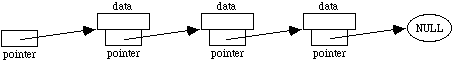In our example, we will describe how to implement a linked list in which the data at each node is a single word (i.e. string of characters). The first task is to define a node. To do this, we can associate a string with a pointer using a structure definition:

```	struct Node
{
char word[MAX_WORD_LENGTH];
Node *ptr_to_next_node;
};
```

or alternatively

```	struct Node;
typedef Node *Node_ptr;

struct Node
{
char word[MAX_WORD_LENGTH];
Node_ptr ptr_to_next_node;
};
```

(Note the semicolon after the "}".) The word "struct" is a reserved word in C++ (analogous to the notion of a record in Pascal). In the first line of the alternative (second) definition of a node above, "Node" is given an empty definition. This is a bit like a function declaration - it signals an intention to define "Node" in detail later, and in the mean time allows the identifier "Node" to be used in the second "typedef" statement.

### The "." and "->" Operators

Having defined the structure "Node", we can declare variables of this new type in the usual way:

```	Node my_node, my_next_node;
```

The values of the (two) individual components of "my_node" can be accessed and assigned using the dot "." operator:

```	cin >> my_node.word;
my_node.ptr_to_next_node = &my_next_node;
```

In the case that pointers to nodes have been declared and assigned to nodes as follows:

```	Node_ptr my_node_ptr, another_node_ptr;
my_node_ptr = new Node;
another_node_ptr = new Node;
```

we can either use dot notation for these types of statement:

```	cin >> (*my_node_ptr).word;
(*my_node_ptr).ptr_to_next_node = another_node_ptr;
```

or write equivalent statements using the "->" operator:

```	cin >> my_node_ptr->word;
my_node_ptr->ptr_to_next_node = &my_next_node;
```

In other words, "my_node_ptr->word" simply means "(*my_node_ptr).word".

Below is a function which allows a linked list to be typed in at the keyboard one string at a time, and which sets the node pointer "a_list" to point to the head (i.e. first node) of the new list. Typing a full-stop signals that the previous string was the end of the list.

```	void assign_list(Node_ptr &a_list)
{
Node_ptr current_node, last_node;

assign_new_node(a_list);
cout << "Enter first word (or '.' to end list): ";
cin >> a_list->word;
if (!strcmp(".",a_list->word))
{
delete a_list;
a_list = NULL;
}
current_node = a_list;			/* LINE 13 */

while (current_node != NULL)
{
assign_new_node(last_node);
cout << "Enter next word (or '.' to end list): ";
cin >> last_node->word;
if (!strcmp(".",last_node->word))
{
delete last_node;
last_node = NULL;
}
current_node->ptr_to_next_node = last_node;
current_node = last_node;
}
}
```

Fragment of Program 7.5.1

We will assume that the function "assign_new_node(...)" used in the above definition is exactly analogous to the function "assign_new_int(...)" in Program 7.1.5.

Here's how the function "assign_list(...)" works in words and diagrams. After the line

```	assign_new_node(a_list);
```

The state of the program is: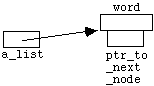Assuming the user now types in "my" at the keyboard, after line 13 the program state is: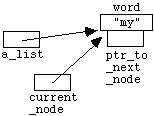After the first line inside the "while" loop, the program state is: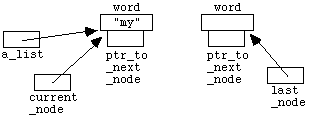Assuming the user now types in "list" at the keyboard, after the "while" loop has finished executing for the first time the situation is: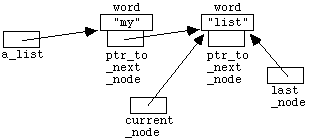After the first line in the second time around the "while" loop, we have: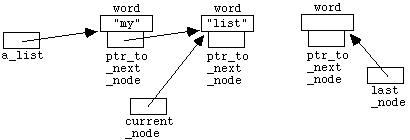Assuming the user now types in "." at the keyboard, after the "while" loop has finished executing for the second time the situation is: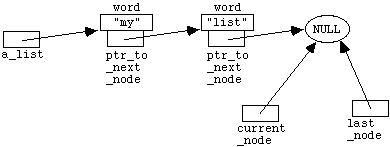Since the condition for entering the "while" loop no longer holds, the function exits, the temporary pointer variables "current_node" and "last_node" (which were declared inside the function body) are automatically deleted, and we are left with: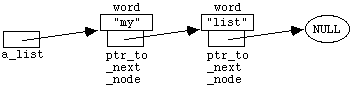Printing our linked lists is straightforward. The following function displays the strings in the list one after another, separated by blank spaces:

```	void print_list(Node_ptr a_list)
{
while (a_list != NULL)
{
cout << a_list->word << " ";
a_list = a_list->ptr_to_next_node;
}
}
```

Second fragment of Program 7.5.1

## 7.6 Summary

In this lecture we have seen how computer memory can be dynamically allocated and de-allocated during the execution of a program, using pointers. We have discussed how arrays may be manipulated using pointer arithmetic, and how arrays may be created and destroyed dynamically by a program. Finally, we have briefly introduced the notion of a linked list, as an example of an abstract data type which may be implemented using pointers. The material here is also covered in more detail in Savitch, Chapter 9 and Chapter 13.